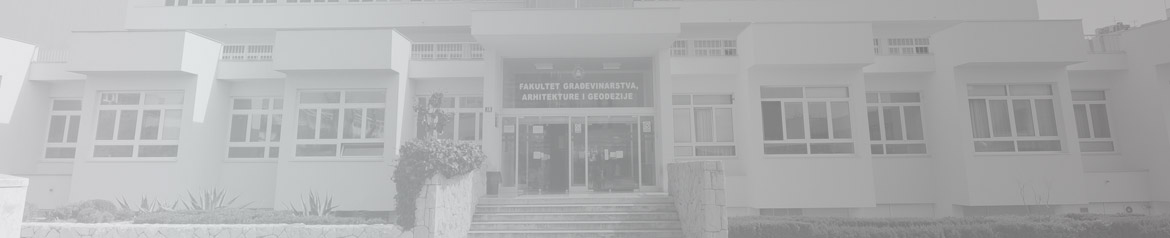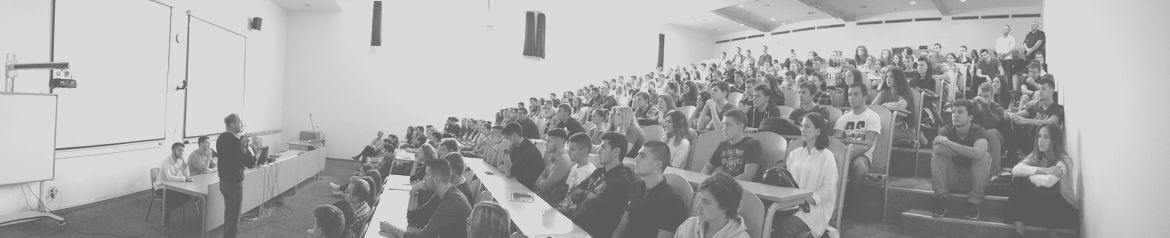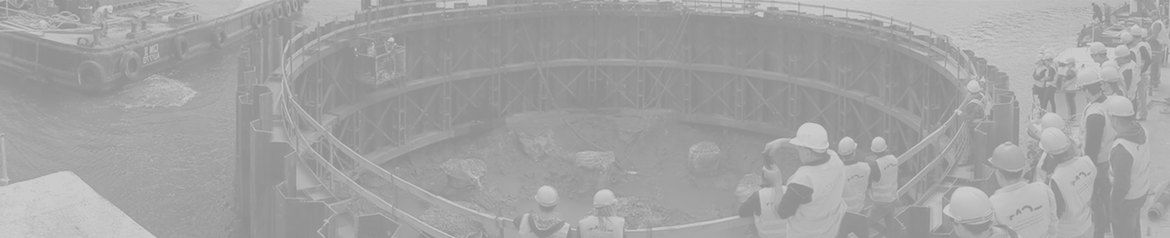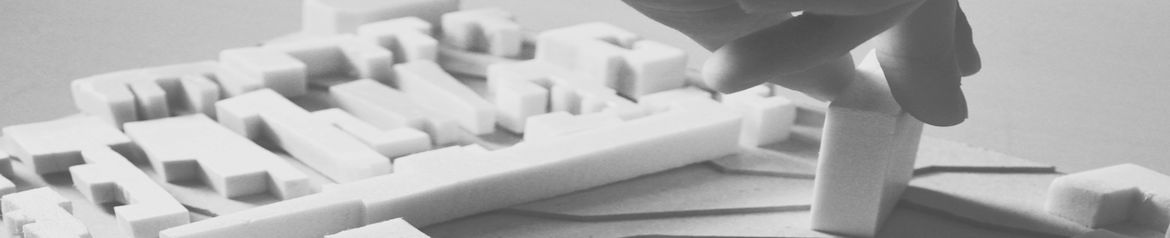•••••# Sveučilište u Splitu - Fakultet građevinarstva, arhitekture i geodezijeUniversity of Split - Faculty of Civil Engineering, Architecture and Geodesy

## Department of Mathematics and Physics

 Study Course Semester Hours ECTS Undergraduate University Study of Civil Engineering Mathematics I Physics Basics of Engineering Informatics Mathematics II Probability and Statistics Introduction to Programming  Applied Mathematics I. I. I. III. II. II. VI. 60+60 45+15 15+45 60+60 30+30 15+30 30+30 10,0 5,0 3,5 10,0 5,0 3,0 5,0 Graduate University Study of Civil Engineering Applied Mathematics I. 30+30 5,0 Postgraduate Doctoral Study of Civil Engineering Applied Functional Analysis Optimization Methods Mathematical Analysis of Boundary Value Problems Integral Equation Methods of Mathematical Statistics 6,0 6,0 6,0 6,0 6,0 Undergraduate University Study of Architecture and Urban Planning Mathematics I Mathematics II I. II. 15+15 15+15 2,0 2,0 Undergraduate University Study of Geodesy and Geoinformatics Analytical Geometry and Linear Algebra Mathematical Analysis Physics Basics of Computer Science Vector Analysis Basics of Statistics Spherical Trigonometry Differential Geometry Discrete Mathematics I. I. I. I. III. II. II. II. VI. 30+30 30+30 30+30 15+15 30+15 30+15 15+15 30+30 30+30 5,0 5,0 5,0 2,0 3,0 4,0 3,0 5,0 5,0 Undergraduate Professional Study of Civil Engineering Mathematics Basics of Engineering Informatics I I. I. 60+60 15+45 9,0 4,0

Learning outcomes:

Undergraduate University Study of Civil Engineering
Mathematics I – students will be able to:
- solve some mathematical equations and inequalities and interpret them graphically;
- compute limits, derivatives, integrals and Taylor series;
- approximate functions by means of Taylor polynomials;
- use derivatives and integrals to sketch a graph of a function, compute the area of a plane figure, compute the volume and area of a solid of revolution, compute the length of a graph;
- solve simple geometric problems with a vector account;
- solve systems of linear equation.

Physics – students will be able to:
- understand the basic physical laws and the basis of geophysics with applications in everyday tasks of geodesy and geoinformatics.

Basics of Engineering Informatics - students will be able to:
- apply basic concepts of operating systems and computer security measures in computer use;
- create seminar work (including tables, formulas, graphical attachments), using a computer program for text processing;
- create a spreadsheet with basic calculations, data analysis, graphing;
- perform symbolic mathematical calculations using a computer: algebraic transformations
expression, derivation, integration, calculation of limes;
- graphically display the functions of a variable;
- make a simple drawing of two-dimensional CAD using a CAD computer
programs.

Mathematics II – students will be able to:
- compute limes, partial derivations, differentials of real functions of multiple variables with geometrically interpretation;
- solve double and triple integral, curve and surface integrals with applications;
- define and compute the concepts of scalar and vector fields, differential gradient operators, divergence and rotation on appropriate fields with applications;
- solve more important types of differential equations of the first and second order with initial conditions with applications;
- Solve some systems of ordinary differential equations.

Probability and Statistics – students will be able to:
- apply basic combinatorial and probabilistic methods for solving combinatorial and probability problems;
- apply one-dimensional and two-dimensional discrete and one-dimensional continuous distributions to solve practical probability problems;
- analyze statistical data through frequencies, sample function of distribution and estimation of unknown parameters;
- define parameter estimates, their distribution, confidence intervals, and test hypotheses about parameters of the given random variables;
- regulate correlation and regression between random variables based on the sample.

Introduction to Programming - students will be able to:
- create a simple program flow diagram based on the default program task;
- write and run a simple computer program (using FORTRAN 90 programming language);
- use data types (integer, real, logical, sign) in solving program task;
- use control commands (trimming programs, loops) in solving the program task;
- use text input and output files;
- write and implement a program for applying simple numerical mathematics methods;
- use software modules to create a computer program;
- create a simple macro program using Visual Basic.

Applied mathematics - students will be able to:
- develop a function in Fourier's series and polynomials;
- describe and solve some boundary problems of partial differential equations;
- calculate the numerical value of a function and a given integer;
- numerically solve the nonlinear algebraic equation and system of linear algebraic equations;
- describe the least squares method and determine the empirical function for a series of measurements;
- determine approximate solutions to the initial and marginal problems of ordinary differential equations.

Graduate University Study of Civil Engineering
Applied mathematics - students will be able to:
- develop a function in Fourier's series and polynomials;
- describe and solve some boundary problems of partial differential equations;
- calculate the numerical value of a function and a given integer;
- numerically solve the nonlinear algebraic equation and system of linear algebraic equations;
- describe the least squares method and determine the empirical function for a series of measurements;
- determine approximate solutions to the initial and marginal problems of ordinary differential equations.

Postgraduate Doctoral Study of Civil Engineering
Applied Functional Analysis
– students will be able to:
- apply variational approximation of boundary value problems;
- establish the existence and uniqueness of the weak solutions of some boundary value problems;
- to examine the solvency conditions of linear algebraic and operator equations.

Optimization Methods – students will be able to:
- assess whether a practical problem can be formulated as a problem of mathematical optimization;
- to argue whether the mathematical optimization problem formulated is one of the class of problems that can be reliably and efficiently solved by optimization methods (least squares method, linear programming, convex optimization);
- develop algorithms to solve moderate size problems with the optimization method chosen;
- characterize the optimum solution.

Mathematical Analysis of Boundary Value Problems – students will be able to:
- formulate partial differential equations for the given physical problems;
- classify partial differential equations in linear, quasilinear and nonlinear;
- evaluate whether the partial differential equation can be solved by analytical methods and select the appropriate method;
- evaluate whether the partial partial differential equation can be solved by numerical methods and select the appropriate method.

Integral Equation - students will be able to:
- formulate integral equations for solving initial and boundary value problems of ordinary and partial differential equations;
- classify the integral equation and choose an appropriate method of solving;
- determine whether or not to apply the application of integral transformation;
- determine whether numerical methods can be applied in solving integral equations.

Methods of Mathematical Statistics – students will be able to:
- formulate a stochastic model of practical problems with an emphasis on water management;
- choose a statistical method or model evaluation test;
- evaluate the obtained results of the formed stochastic model;
- evaluate the constraints of the selected model.

Undergraduate University Study of Architecture and Urban Planning
Mathematics I – students will be able to:
- solve some basic problems from analytic geometry;
- explain and graphically illustrate the basic concepts and properties of the real function of the one variable;
- define the concepts and compute limits and derivatives of real functions of one variable;
- use derivatives to sketch a graph of a function.

Mathematics II – students will be able to:
- calculate an indefinite, definite and improper integral;
- use integrals to compute the area of a plane figure, compute the volume and area of a solid of revolution, compute the length of a graph;
- solve some basic  differential equations.

Undergraduate University Study of Geodesy and Geoinformatics
Analytical geometry and linear algebra – students will be able to:
- solve some geometric problems with vectors;
- solve mathematical equation and linear equation systems;
- determine basis, dimension, subspace of some vector spaces;
- determine operator linearity;
- determine the characteristic equation, eigenvalues and eigenvectors for linear operators;
- classify curves and planes of the second order.

Mathematical analysis – students will be able to:
- solve some mathematical equations and inequalities and interpret them graphically;
- compute limits, derivatives, integrals;
- use derivatives and integrals to sketch a graph of a function, compute the area of a plane figure, compute the volume and area of a solid of revolution, compute the length of a graph;
- solve more important types of differential equations with applications.

Physics – students will be able to:
- understand the basic physical laws and the basis of geophysics with applications in everyday tasks of geodesy and geoinformatics.

Basics of Computer Science – students will be able to:
- apply basic concepts of operating systems and computer security measures in computer use;
- create seminar work (including tables, formulas, graphical attachments), using a computer program for text processing;
- create a spreadsheet with basic calculations, data analysis, graphing;
- perform symbolic mathematical calculations using a computer: algebraic transformations
expression, derivation, integration, calculation of limes;
- graphically display the functions of a variable;
- make a simple drawing of two-dimensional CAD using a CAD computer
programs.

Vector analysis – students will be able to:
- define the concepts and compute limes, partial derivations, differentials of real functions of multiple variables with geometrically interpretation;
- solve double and triple integral, curve and surface integrals with applications;
- define and compute the concepts of scalar and vector fields, differential gradient operators, divergence and rotation on appropriate fields with applications.

Basics of Statistics – students will be able to:
- apply basic combinatorial and probabilistic methods for solving combinatorial and probability problems;
- apply one-dimensional and two-dimensional discrete and one-dimensional continuous distributions to solve practical probability problems;
- analyze statistical data through frequencies, sample function of distribution and estimation of unknown parameters;
- define parameter estimates, their distribution, confidence intervals, and test hypotheses about parameters of the given random variables;
- regulate correlation and regression between random variables based on the sample.

Spherical trigonometry – students will be able to:
- use basic concepts related to spherical trigonometry;
- distinguish the relations among the main geometrical elements on the sphere;
- use spherical coordinate systems;
- apply spherical geometry to problems in geodesy and astronomy.

Differential geometry – students will be able to:
- determine the length of the arc, singular point, Frenet's formula, flex and torsion of
parameterized curves with geometrical interpretation;
- classify the curve based on the known flexion and torsion;
- determine the coordinate vectors, the tangential plane, and the normal parameterized surface;
- determine the length of the arc of the curve, the angle between the curve on the surface and the surface using the first fundamental form;
- classify the points on the plate by calculating the Gaussian and middle curvature with geometrical interpretation.

Discrete mathematics – students will be able to:
- apply counting principles on simpler practical problems;
- design and simplify logical circuits and networks using Boolean algebra;
- resolve some of the most common practical problems (the travelling salesman problem, shortest path problem, the consistent naming of a graph) by applying graph theory;
-determine algorithms for solving some of the most common practical problems (nearest neighbor algorithm, spanning tree algorithm, topological sort algorithm, Warshall algorithm) by applying graph theory;
- evaluate the efficiency of some simpler algorithms.

Undergraduate Professional Study of Civil Engineering
Mathematics – students will be able to:
- solve some mathematical equations and inequalities and interpret them graphically;
- compute limits, derivatives, integrals;
- use derivatives and integrals to sketch a graph of a function, compute the area of a plane figure, compute the volume and area of a solid of revolution, compute the length of a graph;
- solving systems of linear equations by Gaussian elimination;
- solve more important types of differential equations of the first and second order with initial conditions with applications.

Basics of Engineering Informatics I - students will be able to:
- apply basic concepts of operating systems and computer security measures in computer use;
- create seminar work (including tables, formulas, graphical attachments), using a computer program for text processing;
- create a spreadsheet with basic calculations, data analysis, graphing;
- perform symbolic mathematical calculations using a computer: algebraic transformations
expression, derivation, integration, calculation of limes;
- graphically display the functions of a variable;
- make a simple drawing of two-dimensional CAD using a CAD computer
programs.